# It is a question of studying the dynamic behavior of landing gear (LG) of a F18 landing. To simulate the behavior of the...

```It is a question of studying the dynamic behavior of landing gear (LG) of a F18 landing.
To simulate the behavior of the system we use the figure below.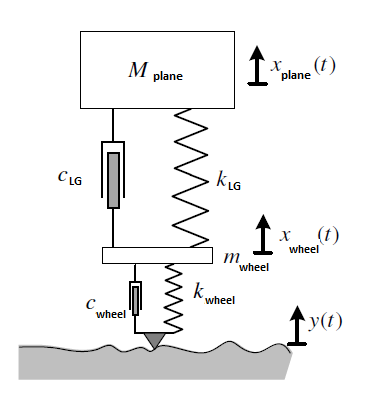The term 'mwheel' denotes the total mass of the constituent members of the wheel.
We want to know the displacement 'xplane(t)' of the mass 'mplane' for different scenarios.
We consider that the state of the airstrips can be represented by an entry 'y(t)' which simulates the
real geometry of the roadway. The tire is modeled by a linear wheel k and a shock absorber
wheel c.
1. Establish the dynamic equations of system operation;
2. Model the detailed block diagram of the system;
3. Deduce the expression of the transfer function: H(s)= Xplane(s)/Y(s) , the initial conditions
being assumed to be zero;
```
.(t) M plane x plane CLG (t) wheel wheel k. 'wheel wheel y(t) www

In this solution some basic concepts and formulas of Vibration Mechanics are used. For more information, refer to any standard textbook or drop a comment below. Please give a Thumbs Up, if solution is helpful.

Solution :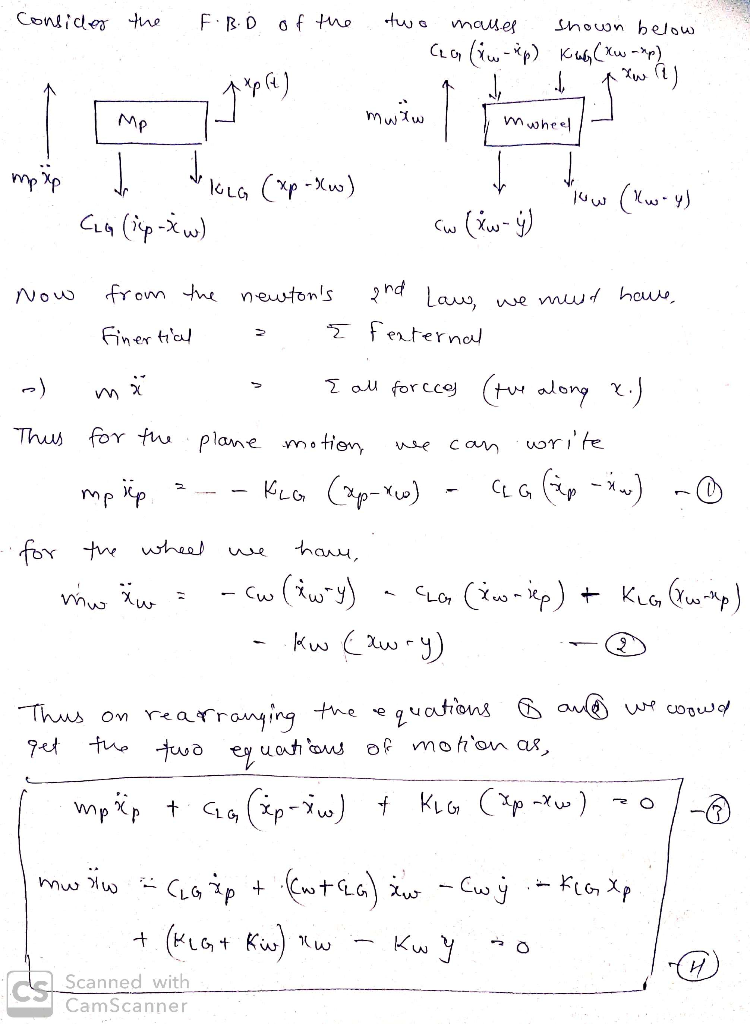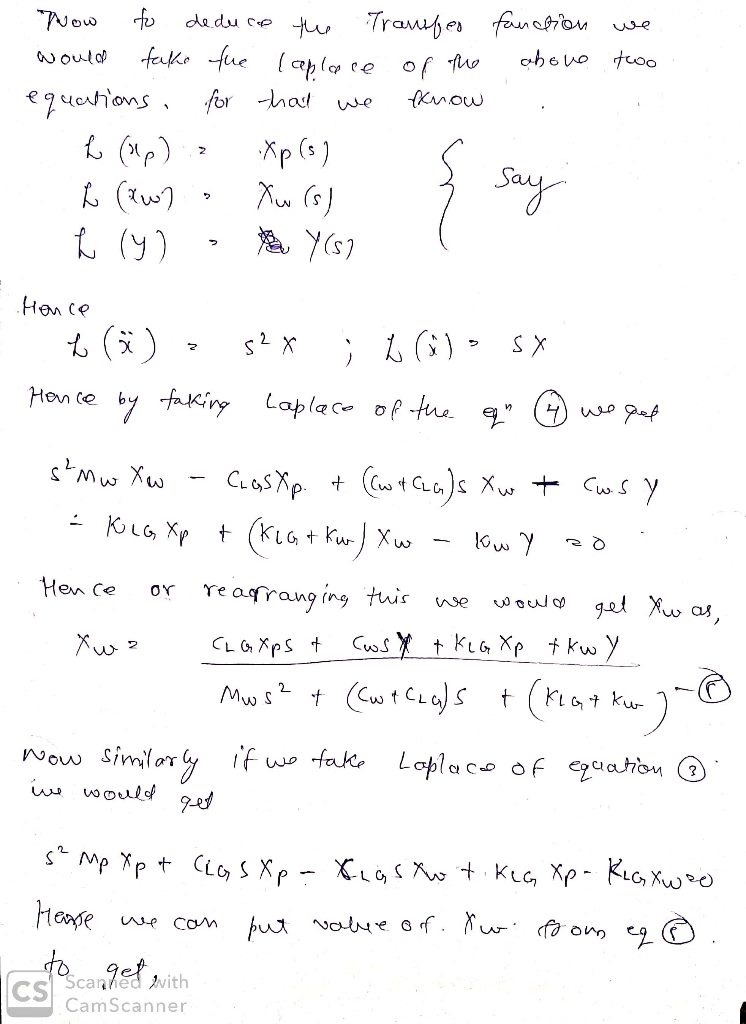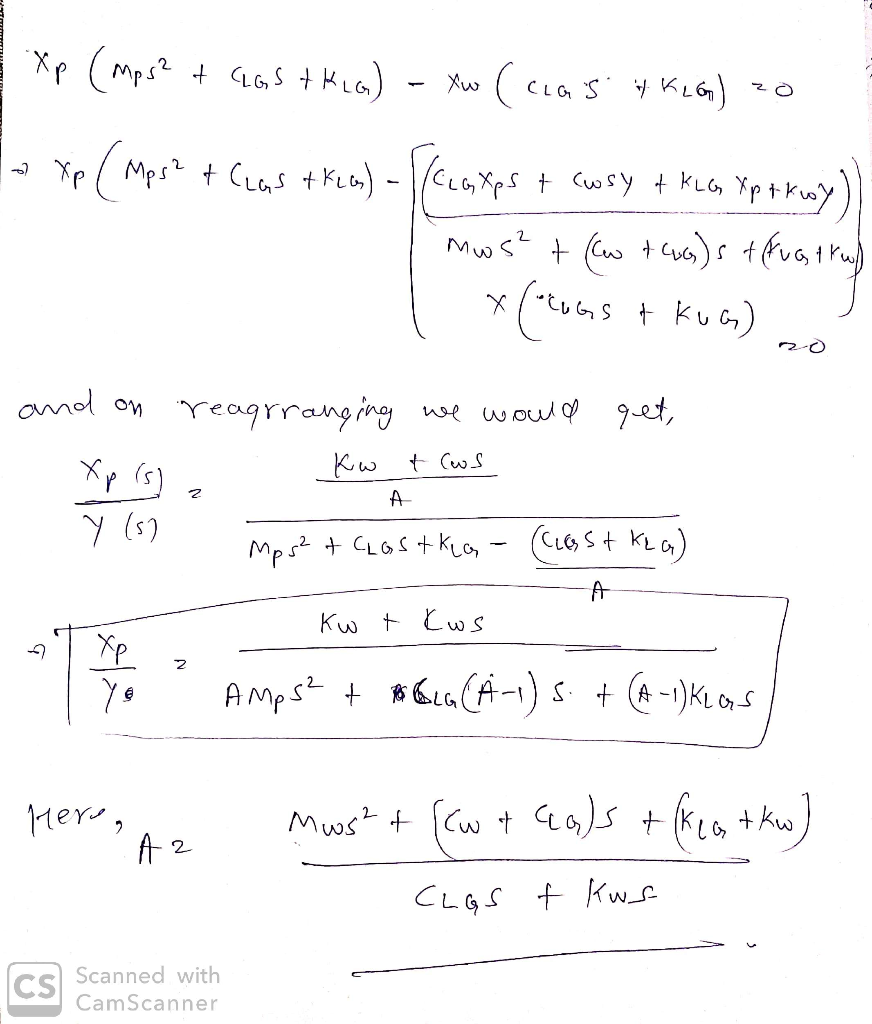NOTE : Feel free to ask further queries. Your positive rating would be appreciated and motivate me !

##### Add Answer of: It is a question of studying the dynamic behavior of landing gear (LG) of a F18 landing. To simulate the behavior of the...
Similar Homework Help Questions
• ### .matlab Objective: This activity has the purpose of helping students to to use either Simulink or VisSim to simulate the system behavior based on its Block Diagram representation and plot its resp...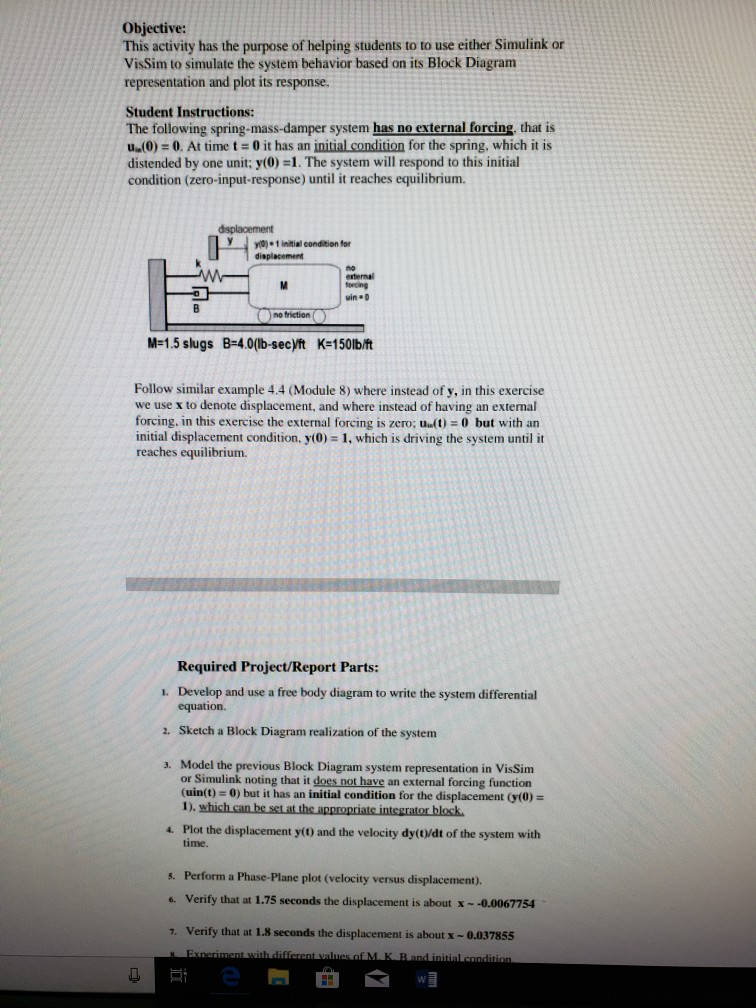.matlab Objective: This activity has the purpose of helping students to to use either Simulink or VisSim to simulate the system behavior based on its Block Diagram representation and plot its response. Student Instructions: The following spring-mass-damper system has no external forcing, that is u(0)-0. At time t- 0 it has an initial condition for the spring, which it is distended by one unit: y(0)-1. The system will respond to this initial condition (zero-input-response) until it reaches equilibrium. 0)1initial condition...

• ### An engineering company is designing and testing a car suspension system. The system has a convent...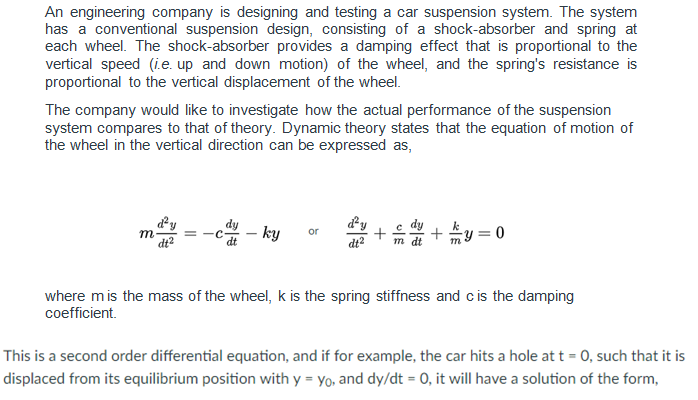Create a MATLAB script An engineering company is designing and testing a car suspension system. The system has a conventional suspension design, consisting of a shock-absorber and spring at vertical speed (ie. up and down motion) of the wheel, and the spring's resistance is proportional to the vertical displacement of the wheel. The company would like to investigate how the actual performance of the suspension system compares to that of theory. Dynamic theory states that the equation of motion of...

• ### Objective: This activity has the purpose of helping students to to use either Simulink or VisSim to simulate the system behavior based on its Block Diagram representation and plot its response...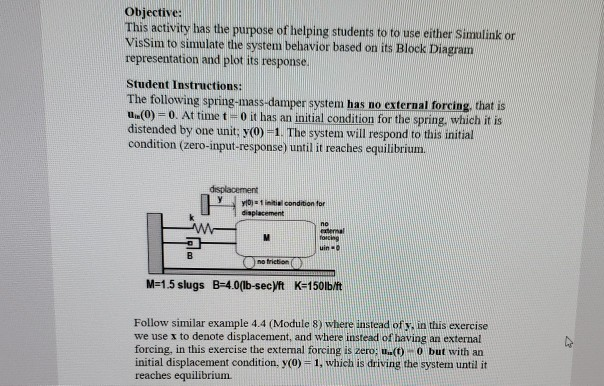Objective: This activity has the purpose of helping students to to use either Simulink or VisSim to simulate the system behavior based on its Block Diagram representation and plot its response Student Instructions: The following spring-mass-damper system has no external forcing, that is Lu(0) 0. At time t"0 it has an initial condition for the spring, which it is distended by one unit; yO) 1. The system will respond to this initial condition (zero-input-response) until it reaches equilibriunm. | yin«...

• ### An engineering company is designing and testing a car suspension system. The system has a convent...

An engineering company is designing and testing a car suspension system. The system has a conventional suspension design, consisting of a shock-absorber and spring at each wheel. The shock-absorber provides a damping effect that is proportional to the vertical speed (i.e. up and down motion) of the wheel, and the spring's resistance is proportional to the vertical displacement of the wheel. The design team analyses the suspension system in two separate parts: Part 1 - dynamics of the spring-damper system;...

• ### An engineering company is designing and testing a car suspension system. The system has a convent...An engineering company is designing and testing a car suspension system. The system has a conventional suspension design, consisting of a shock-absorber and spring at each wheel. The shock-absorber provides a damping effect that is proportional to the vertical speed (i.e. up and down motion) of the wheel, and the spring's resistance is proportional to the vertical displacement of the wheel.The design team analyses the suspension system in two separate parts: Part 1 - dynamics of the spring-damper system; Part 2...

• ### Circular Motion and torque Question (super long)

x.øi5ze="2">Questions in this exam are numbered in the format of 'n:' or 'n:c' wheren is a number. For questions numbered as 'n:c', your answer should beone of the keys (A-AC) in this list of choices.A: south-eastB: northC: undefinedD: north-westE: southF: westG: south-westH: eastI: north-eastJ: falseK: trueL: smallerM: greaterN: a stable equilibrium stateO: a non-equilibrium stateP: an unstable equilibrium stateQ: canR: cannotS: increaseT: decreaseU: anti-parallelV: parallelW: perpendicularX: isY: is notZ: frictional forceAA: normal forceAB: accelerateAC: decelerate==== Problem 1 (15 points total)...

Need Online Homework Help?